# [ 03: Linear Algebra – Review ]

Video Lectures of Matrices
Matrices – overview
• Rectangular array of numbers written between square brackets
• 2D array
• Named as capital letters (A,B,X,Y)
• Dimension of a matrix are [Rows x Columns]
• Start at top left
• To bottom left
• To bottom right
• R[r x c] means a matrix which has r rows and c columns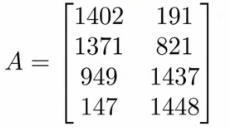• Is a [4 x 2] matrix
• Matrix elements
• A(i,j) = entry in ith row and jth column• Provides a way to organize, index and access a lot of data
Vectors – overview
• Is an n by 1 matrix
• Usually referred to as a lower case letter
• n rows
• 1 column
• e.g.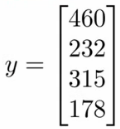• Is a 4 dimensional vector
• Refer to this as a vector R4
• Vector elements
• vi = ith element of the vector
• Vectors can be 0-indexed (C++) or 1-indexed (MATLAB)
• In math 1-indexed is most common
• But in machine learning 0-index is useful
• Normally assume using 1-index vectors, but be aware sometimes these will (explicitly) be 0 index ones
Matrix manipulation
• Addition
• Add up elements one at a time
• Can only add matrices of the same dimensions
• Creates a new matrix of the same dimensions of the ones added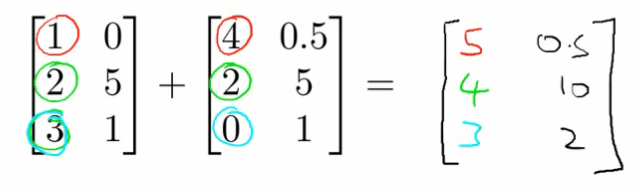• Multiplication by scalar
• Scalar = real number
• Multiply each element by the scalar
• Generates a matrix of the same size as the original matrix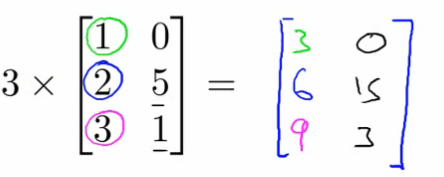• Division by a scalar
• Same as multiplying a matrix by 1/4
• Each element is divided by the scalar
• Combination of operands
• Evaluate multiplications first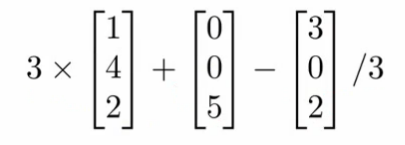• Matrix by vector multiplication
• [3 x 2] matrix * [2 x 1] vector
• New matrix is [3 x 1]
• More generally if [a x b] * [b x c]
• Then new matrix is [a x c]
• How do you do it?
• Take the two vector numbers and multiply them with the first row of the matrix
• Then add results together – this number is the first number in the new vector
• The multiply second row by vector and add the results together
• Then multiply final row by vector and add them together• Detailed explanation
• A * x = y
• A is m x n matrix
• x is n x 1 matrix
• n must match between vector and matrix
• i.e. inner dimensions must match
• Result is an m-dimensional vector
• To get yi – multiply A’s ith row with all the elements of vector x and add them up
• Neat trick
• Say we have a data set with four values
• Say we also have a hypothesis hθ(x) = -40 + 0.25x
• Create your data as a matrix which can be multiplied by a vector
• Have the parameters in a vector which your matrix can be multiplied by
• Means we can do
• Prediction = Data Matrix * Parameters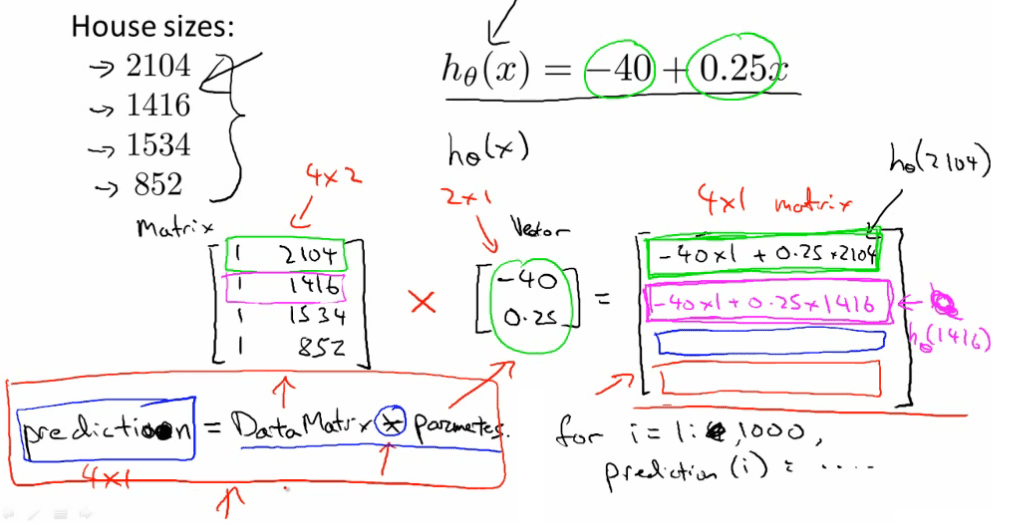• Here we add an extra column to the data with 1s – this means our θvalues can be calculated and expressed
• The diagram above shows how this works
• This can be far more efficient computationally than lots of for loops
• This is also easier and cleaner to code (assuming you have appropriate libraries to do matrix multiplication)
• Matrix-matrix multiplication
• General idea
• Step through the second matrix one column at a time
• Multiply each column vector from second matrix by the entire first matrix, each time generating a vector
• The final product is these vectors combined (not added or summed, but literally just put together)
• Details
• A x B = C
• A = [m x n]
• B = [n x o]
• C = [m x o]
• With vector multiplications o = 1
• Can only multiply matrix where columns in A match rows in B
• Mechanism
• Take column 1 of B, treat as a vector
• Multiply A by that column – generates an [m x 1] vector
• Repeat for each column in B
• There are o columns in B, so we get o columns in C
• Summary
• The i th column of matrix C is obtained by multiplying A with the th column of B
• Start with an example
• A x B• Initially
• Take matrix A and multiply by the first column vector from B
• Take the matrix A and multiply by the second column vector from B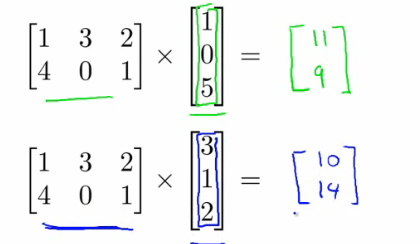• 2 x 3 times 3 x 2 gives you a 2 x 2 matrix
Implementation/use
• House prices, but now we have three hypothesis and the same data set
• To apply all three hypothesis to all data we can do this efficiently using matrix-matrix multiplication
• Have
• Data matrix
• Parameter matrix
• Example
• Four houses, where we want to predict the prize
• Three competing hypotheses
• Because our hypothesis are one variable, to make the matrices match up we make our data (houses sizes) vector into a 4×2 matrix by adding an extra column of 1s• What does this mean
• Can quickly apply three hypotheses at once, making 12 predictions
• Lots of good linear algebra libraries to do this kind of thing very efficiently
Matrix multiplication properties
• Can pack a lot into one operation
• However, should be careful of how you use those operations
• Some interesting properties
• Commutativity

• When working with raw numbers/scalars multiplication is commutative
• 3 * 5 == 5 * 3
• This is not true for matrix
• A x B != B x A
• Matrix multiplication is not commutative
• Associativity
• 3 x 5 x 2 == 3 x 10 = 15 x 2
• Associative property
• Matrix multiplications is associative
• A x (B x C) == (A x B) x C
• Identity matrix
• 1 is the identity for any scalar
• i.e. 1 x z = z
• for any real number
• In matrices we have an identity matrix called I
• Sometimes called I{n x n}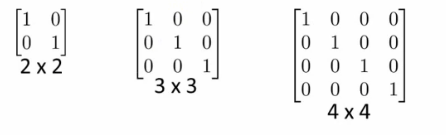• See some identity matrices above
• Different identity matrix for each set of dimensions
• Has
• 1s along the diagonals
• 0s everywhere else
• 1×1 matrix is just “1”
• Has the property that any matrix A which can be multiplied by an identity matrix gives you matrix A back
• So if A is [m x n] then
• A * I
• I = n x n
• I * A
• I = m x m
• (To make inside dimensions match to allow multiplication)
• Identity matrix dimensions are implicit
• Remember that matrices are not commutative AB != BA
• Except when B is the identity matrix
• Then AB == BA
Inverse and transpose operations
• Matrix inverse
• How does the concept of “the inverse” relate to real numbers?
• 1 = “identity element” (as mentioned above)
• Each number has an inverse
• This is the number you multiply a number by to get the identify element
• i.e. if you have x, x * 1/x = 1
• e.g. given the number 3
•  3 * 3-1 = 1 (the identity number/matrix)
• In the space of real numbers not everything has an inverse
• e.g. 0 does not have an inverse
• What is the inverse of a matrix
• If A is an m x m matrix, then A inverse = A-1
• So A*A-1 = I
• Only matrices which are m x m have inverses
• Square matrices only!
• Example
• 2 x 2 matrix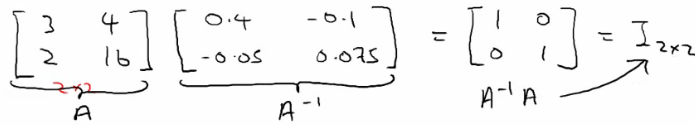• How did you find the inverse
• Turns out that you can sometimes do it by hand, although this is very hard
• Numerical software for computing a matrices inverse
• Lots of open source libraries
• If A is all zeros then there is no inverse matrix
• Some others don’t, intuition should be matrices that don’t have an inverse are a singular matrix or a degenerate matrix (i.e. when it’s too close to 0)
• So if all the values of a matrix reach zero, this can be described as reaching singularity
• Matrix transpose
• Have matrix A (which is [n x m]) how do you change it to become [m x n] while keeping the same values
• i.e. swap rows and columns!
• How you do it;
• Take first row of A – becomes 1st column of AT
• Second row of A – becomes 2nd column…
• A is an m x n matrix
• B is a transpose of A
• Then B is an n x m matrix
• A(i,j) = B(j,i)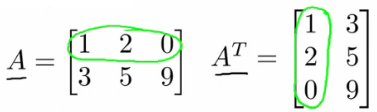## Author: iotmaker

I am interested in IoT, robot, figures & leadership. Also, I have spent almost every day of the past 15 years making robots or electronic inventions or computer programs.